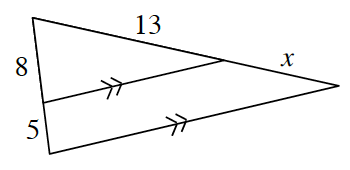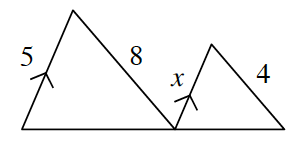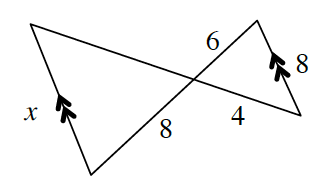### Home > INT2 > Chapter 4 > Lesson 4.1.4 > Problem4-42

4-42.

For each part below, decide if the triangles are similar. If they are similar, use the properties of similarity to solve for $x$. If they are not similar, explain why not.

1.What do the parallel lines tell you about the angles in the triangles?

1.Can you confirm any similar angles?

Not enough information.

1.Which angles are congruent? Which sides correspond?